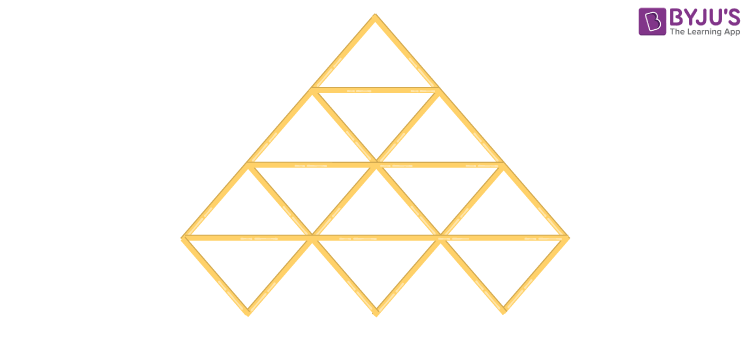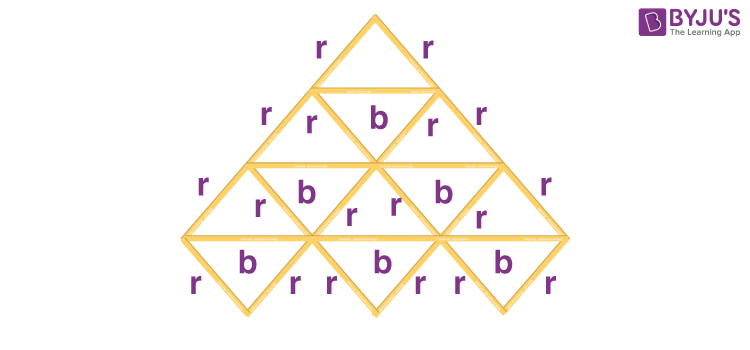# A triangle is made up of 2 red sticks and 1 blue stick. The length of a red stick is given by r and that of a blue stick is given by b. Using this information, write an expression for the total length of sticks in the pattern given

A triangle is made up of 2 red sticks and 1 blue stick. The length of a red stick is given by r and that of a blue stick is given by b. Using this information, write an expression for the total length of sticks in the pattern given:Solution:

Length of red stick = 2r

Length of blue stick = b

Total no.of red stick = 18

Total no.of blue stick = 6

Therefore, total length of stick = 18r+6b=6(3r+b)

Therefore, the expression for the total length of sticks is 6(3r+b)(0)(0)